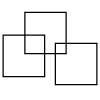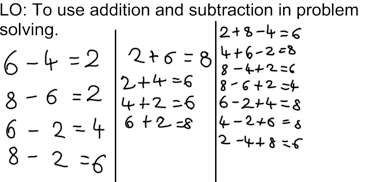#### You may also like### Three Squares

What is the greatest number of squares you can make by overlapping three squares?### Two Dice

Find all the numbers that can be made by adding the dots on two dice.### Biscuit Decorations

Andrew decorated 20 biscuits to take to a party. He lined them up and put icing on every second biscuit and different decorations on other biscuits. How many biscuits weren't decorated?

# 2,4,6,8

##### Age 5 to 7Challenge Level

Jane and Sanjev sent us the following answer:

First we tried to make a statement using the plus on one side of the equals sign and the minus on the other, but we couldn't find one.

Then we tried to use the plus and the minus on the same side, and we got 2 + 8 - 6 = 4.

Then we tried to see what statements we could make starting with the number 2 :

2 + 4 = 6
2 + 6 = 8
2 + 8 - 4 = 6
2 + 8 - 6 = 4

We could not find any more.

4 + 2 = 6
4 + 6 - 8 = 2
4 + 6 - 2 = 8

and with the number 6 :

6 + 2 = 8
6 + 4 - 2 = 8
6 + 4 - 8 = 2

and with the number 8 :

8 + 2 - 4 = 6
8 + 2 - 6 = 4
8 - 2 = 6
8 - 6 = 2

And then we can write them all the other way round, so from 2 + 4 = 6 we get 6 = 2 + 4.

That way we made 28 statements.

We have then had a solution from Amelia Earhart Class Year 2 at Newport C of E Primary School, Isle of WightSome of those look different when the numbers are put in a different order like 2-4+8=6 which you may consider different to 2+8-4=6.
Thank you for your solution from the Isle of Wight.